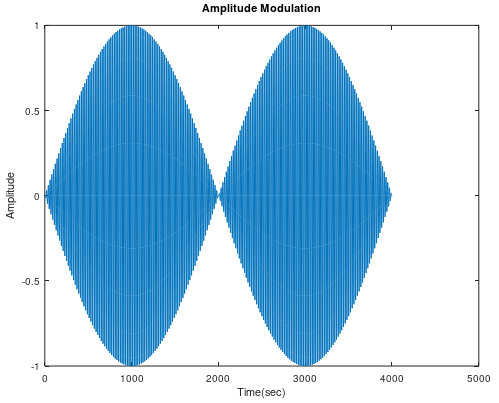Open in App
Not now

# Amplitude Modulation using MATLAB

• Difficulty Level : Medium
• Last Updated : 29 May, 2021

Amplitude modulation (AM) is a modulation technique utilized in electronic communication, most ordinarily for transmitting data by means of a carrier wave. In amplitude modulation, the amplitude that is the signal quality of the carrier wave differs with respect to that of the message signal being transmitted.

Amplitude modulation in MATLAB can be achieved by using the ammod() function.

## ammod()

Syntax : y = ammod(x, Fc, Fs, ini_phase, carramp)
Parameters :

• x : amplitude signal
• Fc : carrier signal frequency
• Fs : sampling frequency
• ini_phase : initial phase in the modulated signal y in radians
• carramp : carrier amplitude of the modulated signal

Returns : amplitude modulated (AM) signal

Example: Amplitude modulation of a sine wave with only 3 parameters.

## MATLAB

 `% carrier Frequency` `Fc = 200; `   `% sampling frequency` `Fs= 4000;`   `% time Duration` `t = (0 : 1 / Fs : 1); `   `% sine Wave with time duration of 't'` `x = sin(2*pi*t);` ` `  `% Amplitude Modulation` `y = ammod(x, Fc, Fs);`   `plot(y);` `title(``'Amplitude Modulation'``);` `xlabel(``'Time(sec)'``);` `ylabel(``'Amplitude'``);`

Output :My Personal Notes arrow_drop_up
Related Articles